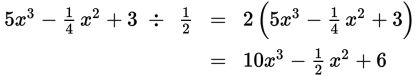# SAT Math Multiple Choice Question 406: Answer and Explanation

### Test Information

Question: 406

1.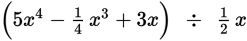What is the result of dividing the two expressions above?

• A.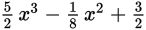• B.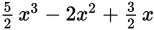• C.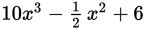• D.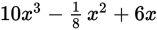Explanation:

C

Difficulty: Medium

Category: Passport to Advanced Math / Exponents

Strategic Advice: Division and factoring are interchangeable, so think of factoring out the x. Then, instead of dividing by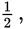you can multiply by its reciprocal, 2. Using these two strategies will make solving a question like this considerably easier.

Getting to the Answer: First, divide (factor) out the x by subtracting 1 from each exponent: The result is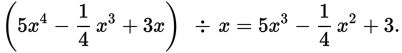Now, multiply each term by 2 to get this: